[BACK]
 Intelligent Automation & Soft ComputingDOI:10.32604/iasc.2022.022606Article

1Chengdu University of Information and Technology, Chengdu, 610255, China
2Southwest Minzu University, Chengdu, 610041, China
3RMIT University, Melbourne, 3058, Australia
*Corresponding Author: Xi Chen. Email: cx@swun.edu.cn
Received: 12 August 2021; Accepted: 08 November 2021

Keywords: Mobile edge computing; deep reinforcement learning; multi-classification; time delay; energy consumption; intelligent inspection swarm; swarm head

1  Introduction

At present, the production and life of human beings are closely linked with weather forecasts, power grids and communication networks. The accuracy of weather forecasts, the safe and stable operation of power grids and communication networks always depend on the normal operation of all kinds of meteorological observation facilities, power facilities and network facilities respectively. Due to the complexity and dynamics of the environment in which various facilities are located (pest tracking) and the diversity of monitoring objects (facility monitoring and biological monitoring), the simple introduction of fixed sensors and cameras may not be able to fully meet the monitoring requirements. Therefore, long-term and effective dynamic monitoring equipment is an important mean to maintain its continuous operation. Intelligent patrol inspection swarm is one of the effective schemes of mobile all-sides monitoring, through the use of multiple low-cost mobile devices (e.g., lightweight drones, smart cars) to construct a unified control, efficient collaboration and dynamic inspection swarm. The swarm nodes dynamically cooperate to complete the monitoring task, transmitting all monitoring data to the cloud, and then returning the result to the control center after processing. Finally, the control center will maintain the equipment according to the result of data processing .

However, there are also some problems in the intelligent inspection swarm. Inspection devices (unmanned aerial vehicles, intelligent cars, etc.) still have defects in battery life and computing power due to the strict constraints of strong mobility, small size and production cost. At the same time, the types of data collected by inspection devices (such as UAVs, intelligent cars, etc.) are diversified with the development of hardware and Internet technology, and the data processing and storage capacity also are enhanced. The data processing mode with cloud computing as the core still has limitations in transmission delay, energy consumption, data security and other aspects [2,3]. Therefore, how to ensure the transmission and processing of data at the minimum cost (delay, energy consumption), and at the same time, maximize swarm’s monitoring time, and then realize the goal to improve the quality of experience in a centralized control the equipment monitoring system. It is one of the key issues to be solved in the intelligent inspection swarm.

Multi-Access Mobile Edge Computing (MEC)  is the key technology to solve this problem. At present, it is widely used in the industrial Internet of Things, autonomous driving, blockchain  and other fields.

A large number of computing tasks are transferred from Mobile wireless devices to nearby access points and executed through connected servers , so as to reduce the energy consumption of devices and the delay and energy consumption of data transmission to the cloud, and effectively ensure data security [5,6].

The rest of this paper is arranged as follows: Section 2 summarizes the relevant work; Section 3 introduces the system model, proposed problem model; Section 4 describes the solution in detail. Section 5 records the experimental simulation results and result’s analysis; Section 6 records the conclusion and future work.

2  Related Work

The development of deep learning has brought a great breakthrough to solve the offloading strategy optimization problem of high-dimensional state and action space in a MEC environment, among which the deep reinforcement learning method is particularly outstanding. The improved DQN and DQN  algorithms are usually applied to the joint optimization of computational offloading and resource allocation in the discrete action space of a single agent. Xiong et al.  proposed that the use of multiple playback memories to store fewer interaction experiences is superior to the original DQN method in terms of convergence and resource allocation. In addition, DDPG [22,23] algorithm performs better than DQN in high and continuous state and action spaces. Chen et al.  designed a dynamic resource management method DDRM based on DDPG, which solved the problems of joint power control of mechatronics and dynamic resource management of computing resource allocation in the industrial Internet of Things. Qian et al.  proposed a joint push and cache strategy based on hierarchical reinforcement learning (HRL). By dividing the total problem into two sub-problems, different basic reinforcement learning methods are adopted to solve the sub-problems.

In general, these works mainly considered the offloading decisions and resource allocation schemes of computation-intensive tasks in the MEC network when a single mobile device offloads tasks to one or more MEC servers , or when multiple mobile devices offload tasks to the same MEC server [10,26]. In the centrally controlled intelligent inspection cluster, considering the high moving frequency of the inspection equipment and the channel state between it and the MEC server, it is necessary to study the computational offloading and resource allocation of all the slave tasks in the cluster determined by a single patrol device.

3  System Model and Problem Formulation

3.1 System Model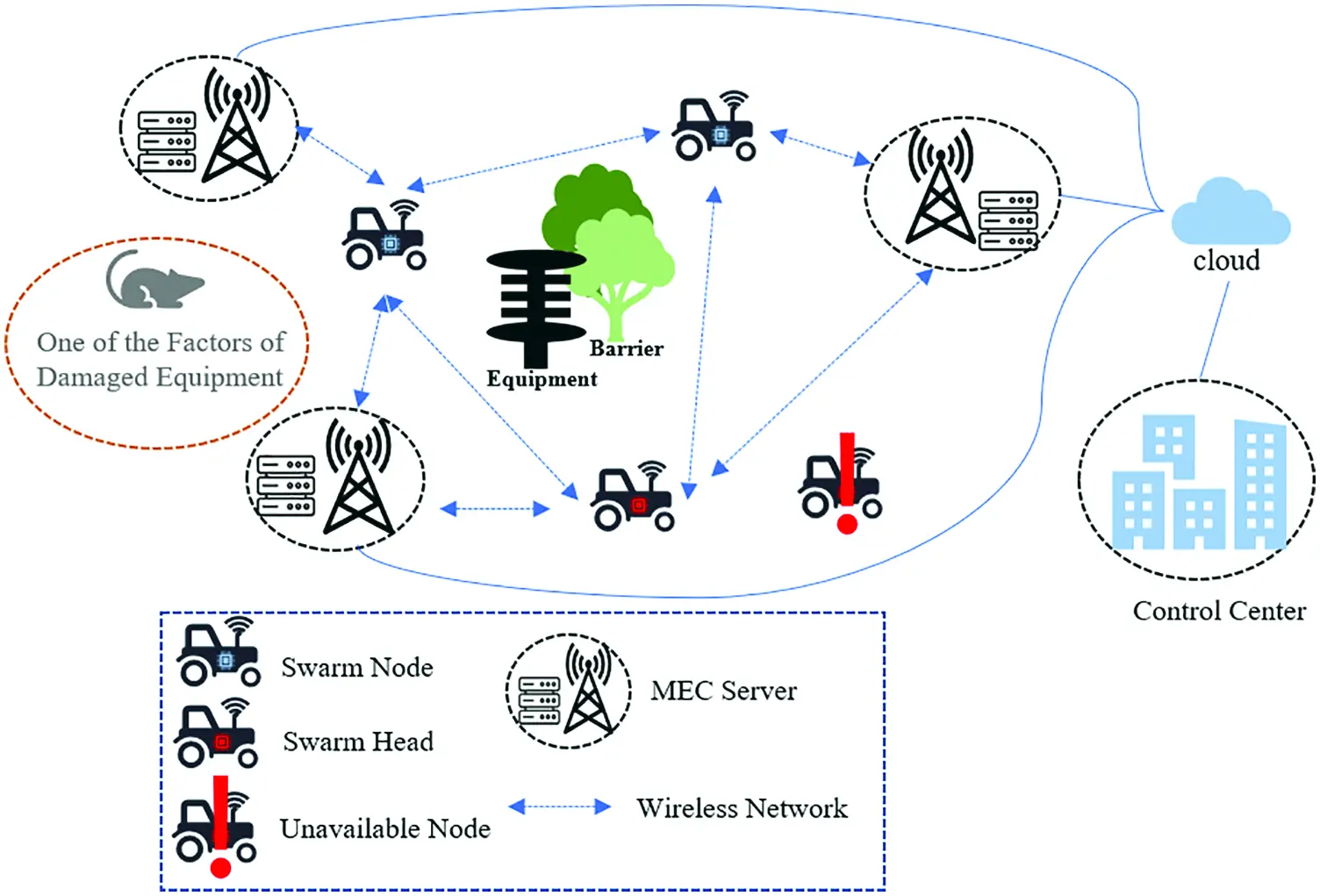Figure 1: MEC system model of intelligent inspection swarm

In each time slot t, the swarm head determines the offloading decision after collecting related information of each slave node. Next, it distributes the decision, and then each node carries out tasks after responding to the decision. The decision of offloading the i-th task of the cluster device n to the edge server w is expressed as xniw(t) ∈ {0, 1}, where w = 0 represents the local computing node, the corresponding value of the selected computing node is 1, and the corresponding value of other nodes is 0, satisfying

wWxniw(t)=1, iI. (1)

constraints. Due to the mobility of inspection devices, the position of cluster devices will change at different time slots t, and the task and channel quality will also change due to the distance change between the devices and BS, thus the offloading decision also should be changed.

3.2 Problem Formulation

3.2.1 Local Computing Model

The swarm inspection device has a certain computing capacity. If the computing resources of the device are sufficient, or the transmission cost of a task is greater than the execution cost of the device itself, the task should be executed locally. Assuming that the delay of the local computing task is only generated by the CPU, and the maximum CPU frequency of the inspection device is expressed in terms of fn,max, the actual computation frequency (In each slot, the execution of different tasks in parallel, and the latency of different tasks is mutually influenced.) is fn, which is the number of CPU cycles running per second while the inspection device is calculating tasks. The delay of the local execution of task i of data device n can be expressed as:

dnilocal(t)=Cni(t)fn(t). (2)

The energy consumption of a single task executed locally can be expressed as:

enilocal(t)=K(fn(t))3dnilocal(t). (3)

where K represents effective switching capacitance in the chip .

3.2.2 Edge Computing Model

rnw(t)=Blog2(1+hnw(t)pnplnw(t)BN0). (4)

where, B represents the communication bandwidth between the device n and the BS w, pn is the transmission power of the device n, N0 is the noise power spectral density of the receiving BS, hnw(t) is the channel gain, plnw(t) the path loss between the device n and the BS w, respectively, where hnw(t)/plnw(t)=g0(Dis0/Disnw(t))σ , g0 is the path loss constant, Dis0 is the reference distance, Disnw is the distance between the device n and the BS w, σ is the path loss exponent. Therefore, the transmission time for the task i generated by device n offloading to the edge server w for processing is denoted as:

dniwtrans(t)=Sni(t)rnw(t). (5)

Let C be the total number of CPUs of the edge server when computing data. The maximum CPU frequency of the edge server is denoted by fw,max, and the actual computing frequency is fnw(t), then the task processing delay in

dniwoff(t)=Cni(t)fnw(t). (6)

The energy consumption of the task i generated by device n offloading to the edge server w for processing can be expressed as:

eniwedge(t)=pndniwtrans(t)+Sni(t)qw. (7)

Here qw is the energy consumed per bit of data on the MEC server.

The delay of the i-th task of device n dni(t) is expressed as:

dni(t)=wW(xniw(t)dnilocal(t)+xniw(t)dniwtrans(t)+xniw(t)dniwoff(t)). (8)

Energy consumption is expressed as:

eni(t)=wW(xniw(t)enilocal(t)+xniw(t)eniwedge(t)). (9)

Therefore, the total time consumption of the whole system in time slot t is:

Dtotal(t)=maxnN,iIdni(t). (10)

Total energy consumption is:

Etotal(t)=nNiIeni(t). (11)

In this paper, considering the balance between improving the QoE of intelligent patrol inspection MEC system, the utilization of cluster computing power and the working time of swarm, the problem of optimizing and minimizing the computing overhead (the weighted average sum of delay and energy consumption) is proposed. Within the time slot t, the computational overhead of all tasks in the cluster is:

Q(t)=αDtotal(t)+βEtotal(t). (12)

where α and β respectively represent the weighted parameters of time and energy consumption required for all tasks, α, β ∈ [0, 1] and α + β = 1. Based on the above formula, at each time slot t, the target optimization problem under the MEC environment of intelligent patrol cluster is expressed as:

P1: min{x}Q(t) (13)

s.t.C1:xniw(t){0,1} (13a)

C2:wWxniw(t)=1 (13b)

C3:iIxniw(t)fn(t)fn,max (13c)

C4:niNIxniw(t)fnw(t)fw,max (13d)

iI,nN,wW

Constraint C1 represents the binary offloading mode; constraint C2 represents that a task can only be offloaded to one node; constraint C3 and C4 represent that the inspection equipment and the edge server need to provide sufficient computing resources for the tasks to be processed at this node, respectively.

4  DDRL and Multi-Classification Based Solution

Reinforcement learning is one of the paradigms and methodologies of machine learning, which is used to describe and solve the problem that agents learn strategies to maximize returns or achieve specific goals in the process of interacting with the environment. The typical model of reinforcement learning is Markov Decision Process (MDP), which generally includes State Space (S), Action Space (A) and Reward Function (R).

In the intelligent inspection MEC system, the task and position of the inspection equipment are time-varying, so problem P1 can be transformed into an MDP problem.

State space: In time slot t, the state space of the system is expressed as:

s(t)={r(t),d(t)} (14)

where, in time slot t, r(t) represents the task information generated by all inspection devices, and d(t) represents the distance information between all inspection devices and BS.

Action space: By observing the current state of the MEC system s(t), we need to determine the offloading plan for each task:

a(t)={xniw(t)|xniw(t){0,1}} (15)

The reward function is defined as a weighted sum of the energy consumption and delay of all devices to complete the task.

re(t)=Q(s(t),a(t)) (16)

With the increase of time slot t, the agent will obtain rewards according to state s(t) selection a(t) , and the expectation of minimizing rewards, namely, the average calculated cost with the weighted sum of time delay and energy consumption as the index, is expressed as follows:

Z(s(t))=E[limT1TT=1Tre(t)] (17)

Therefore, we propose a distributed offloading algorithm, MCDDRL, based on reinforcement learning and multi-classification ideas, for the MEC environment of intelligent inspection swarm. The algorithm structure is shown in Fig. 2 The algorithm consists of two stages: the candidate offloading scheme generation stage and the resource allocation stage. The two stages are completed alternately, and the specific process is as follows.Figure 2: Architecture of MCDDRL algorithm

The algorithm pseudocode is as follows: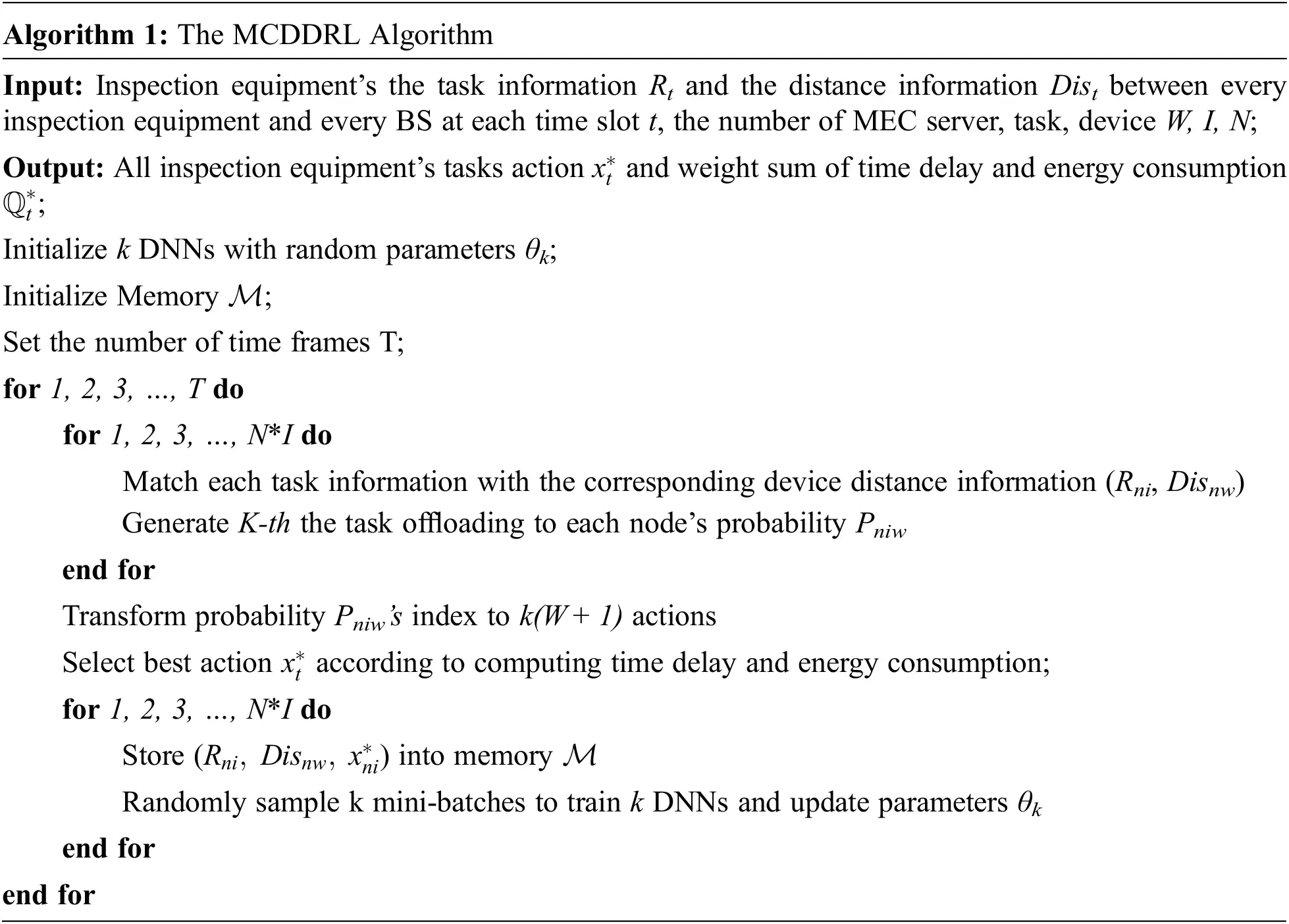Resource allocation stage: firstly, we should calculate the result whether each candidate offloading scheme satisfies constraints C3 and C4 of problem P1; if not, discard that scheme, and then select the one with the smallest value re(t) from the remaining offloading schemes.

After that, the tuple (Rni(t),Disni(t),wWxniw(t)) of each task is stored in a memory pool M . For K DNNs, the learning is carried out by random sampling from the same memory pool respectively, so as to reduce the correlation between samples and update the parameters of DNN k θk by minimizing the cross-entropy loss. In time slot t + 1, the new θk can be used to compute the probability of new tasks being offloaded to the edge server or locally.

5  Numerical Experiment and Result Analysis

In this section, we use simulation experiments to evaluate the performance of MCDDRL algorithm. The experimental platform uses Python 3.8 under Linux system and a deep learning framework TensorFlow-2.1.0-gpu. The experimental parameters and super-parameter settings of MCDDRL algorithm are shown in the Tab. 1, and the super-parameter settings are determined through a large number of experiments.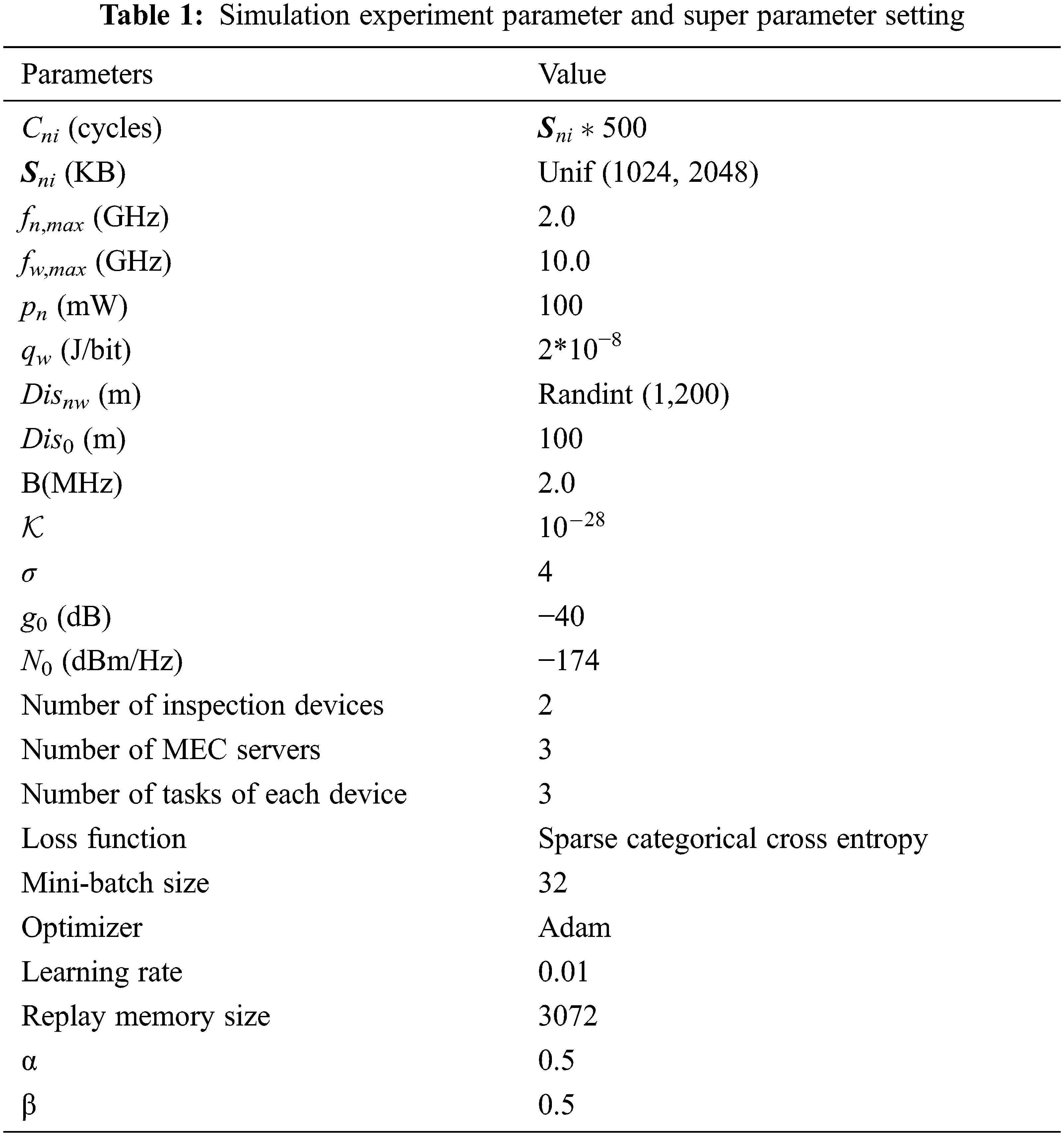To evaluate the influence of parameter K on MCDDRL algorithm, we set the number of K as 1, 5 and 10 respectively, and compare the weighted sum of delay and energy consumption under the different total numbers of time slots. As shown in Fig. 3, when K = 10, the MCDDRL algorithm is superior to K = 1, 5 in terms of time delay and energy consumption weighted sum. The reason is that the more DNNs there are, the more offloading solutions are generated, and the easier it is to find a solution that is close to the optimal solution.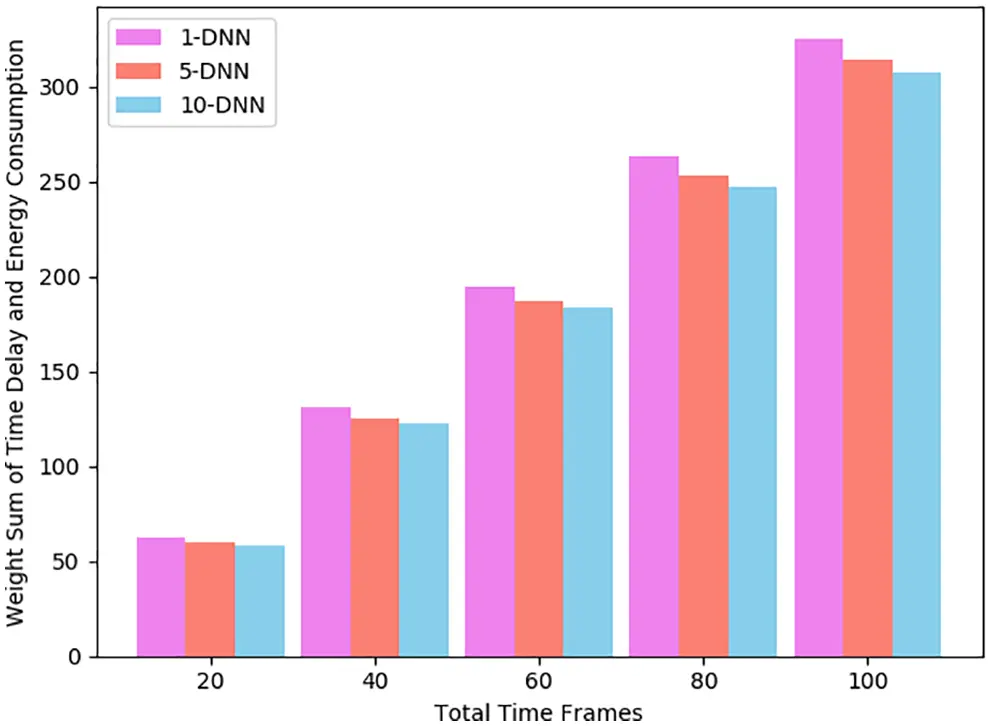Figure 3: Weight sum of time delay and energy consumption under different time frames number for different DNN number

In order to verify the performance of the proposed algorithm, the advantages and disadvantages of the MCDDRL algorithm can be evaluated by comparing the weighted sum of energy consumption and delay, energy consumption and delay of MCDDRL with other offloading algorithms under different time frames number. Comparative offloading algorithms are DDLO  (a distributed algorithm using binary strategy), DQN (Deep Q Network, a reinforcement learning algorithm), random offloading algorithm (ROA), greedy algorithm (It will enumerate all possible schemes and select the weighted sum of the smallest scheme.) and local computing (All tasks are computed in local.).

In this paper, we compare the Time and Energy Weight Sum Reduction Ratio (TEWRR) of MCDDRL, DDLO, ROA and DQN, as shown in Tab. 2. TEWRR represents the value of the difference of the weighted sum of the delay and energy consumption between the above algorithm and local computing and then divided by the weighted sum of local computing. MCDDRL has the highest TEWRR among all algorithms, reaching more than 50%, which is better than DDLO DQN and ROA.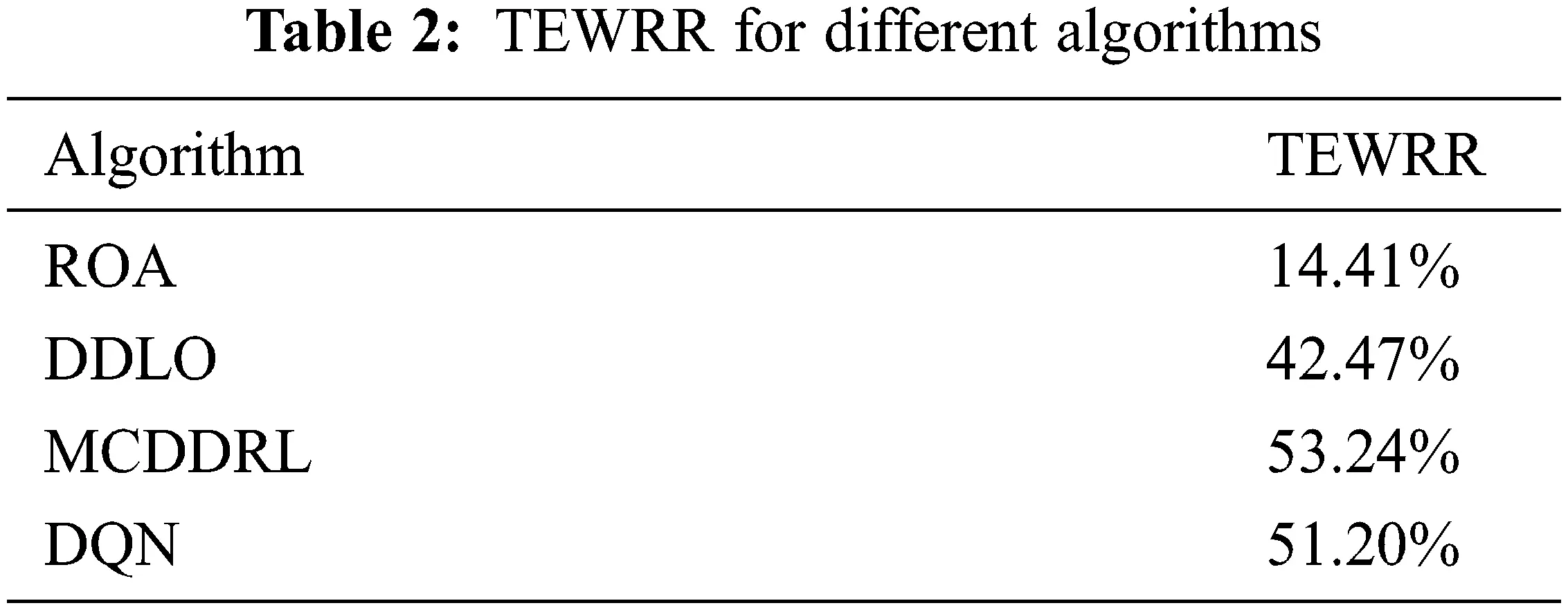We compared the performance of local computing, ROA, greedy algorithm, DDLO, DQN and MCDDRL in terms of the weighted sum of delay and energy consumption, time delay and energy consumption. The value range of total time slot is [0, 400], with 20 as the step size.

In Figs. 46, the time delay, energy consumption and the weighted sum of the two factors show an upward trend with the increase of the number of total time slots, and tasks generated by the MEC system. MCDDRL performs better than DDLO and DQN in the above three aspects and is slightly lower than the greedy algorithm. However, the greedy algorithm is very complicated and time-consuming, so it is not suitable for intelligent the inspection MEC system. At the same time, we found that the energy consumption of the ROA algorithm was lower than that of all other algorithms. Because the amount of task data to be offloading is small result in the total energy consumption of the system is small. Meanwhile, the time delay is far greater than the energy consumption. Therefore, the weighted sum mainly shows the trend of time delay, when α and β are the same. And we can change the dominant position of energy consumption and time delay in the system by adjusting α and β.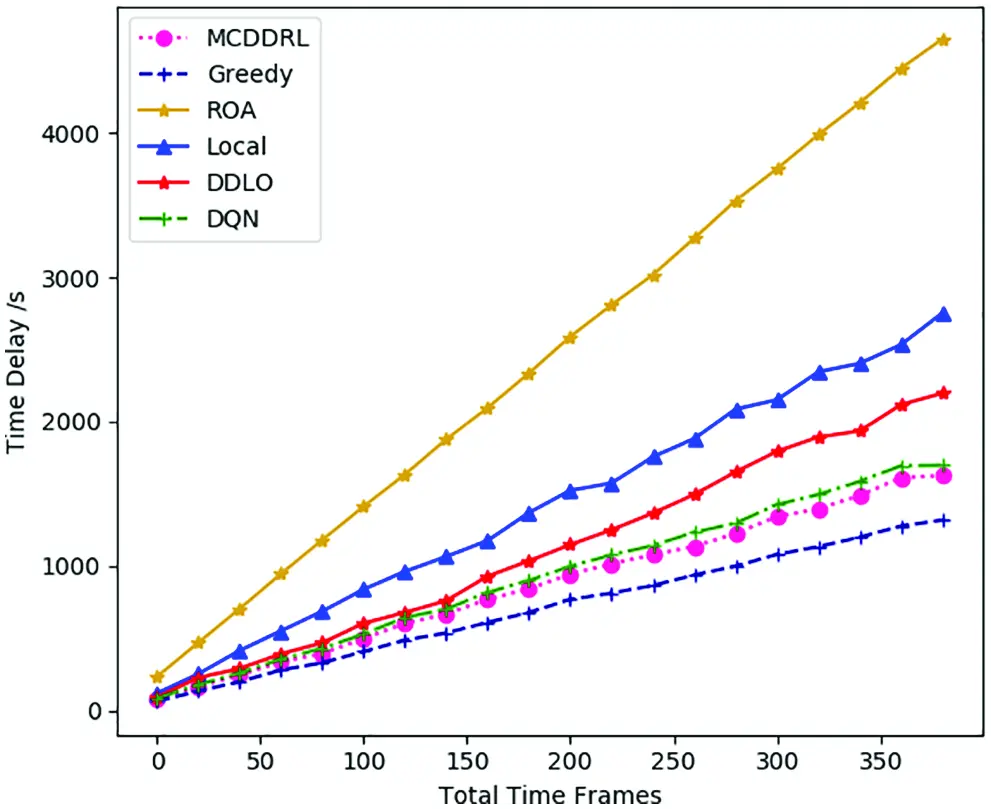Figure 4: Time delay under different time frames number for different algorithms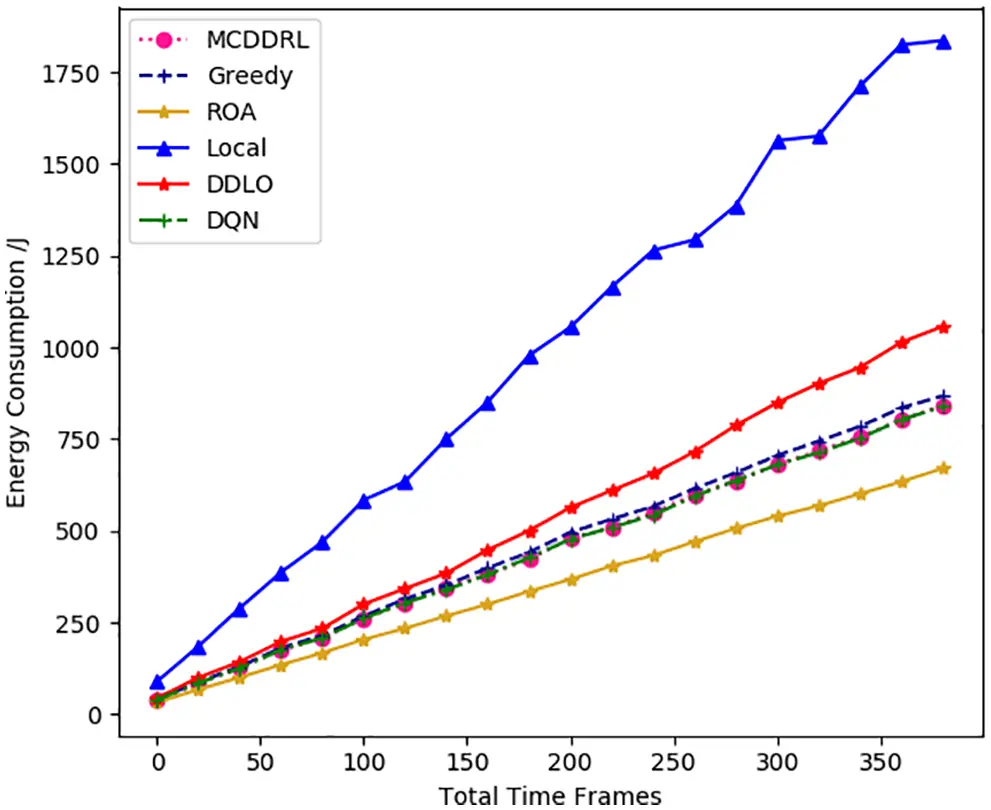Figure 5: Energy consumption under different time frames number for different algorithmsFigure 6: Weight sum of time delay and energy consumption under different time frames number for different algorithms

In Figs. 79, we study the average TEWRR of different edge computing nodes W = [2, 5], inspection devices N = [2, 5] and task number of every device I = [2, 5] under different total time slots. The value range of total time slot is [0, 1600], with 200 as the step size. For MCDDRL, the average value of TEWRR tends to be stable as the total number of time slots increases, no matter W, N, I take any value in the range [2, 5]. In addition, when N and I are fixed, the average value of TEWRR increases as the edge computing node W increases within the range of the same total number of time slots based on the results of Figs. 7 and 10. The reason is that the total number of tasks that need to be processed remains the same in the MEC system, but an increase in W will increase computing resources available in the system, resulting in a positive correlation between the average value of TEWRR and the size of edge computing nodes W. On the contrary, combining the results of Figs. 9 and 10 shows that when W and N are fixed, the average value of TEWRR is inversely proportional to inspection devices I. Because in this case, the available computing resources of the MEC system remain unchanged, and an increase in I will increase the total number of tasks to be processed in each time slot.Figure 7: The average TEWRR of different edge computing nodes W under different total time slots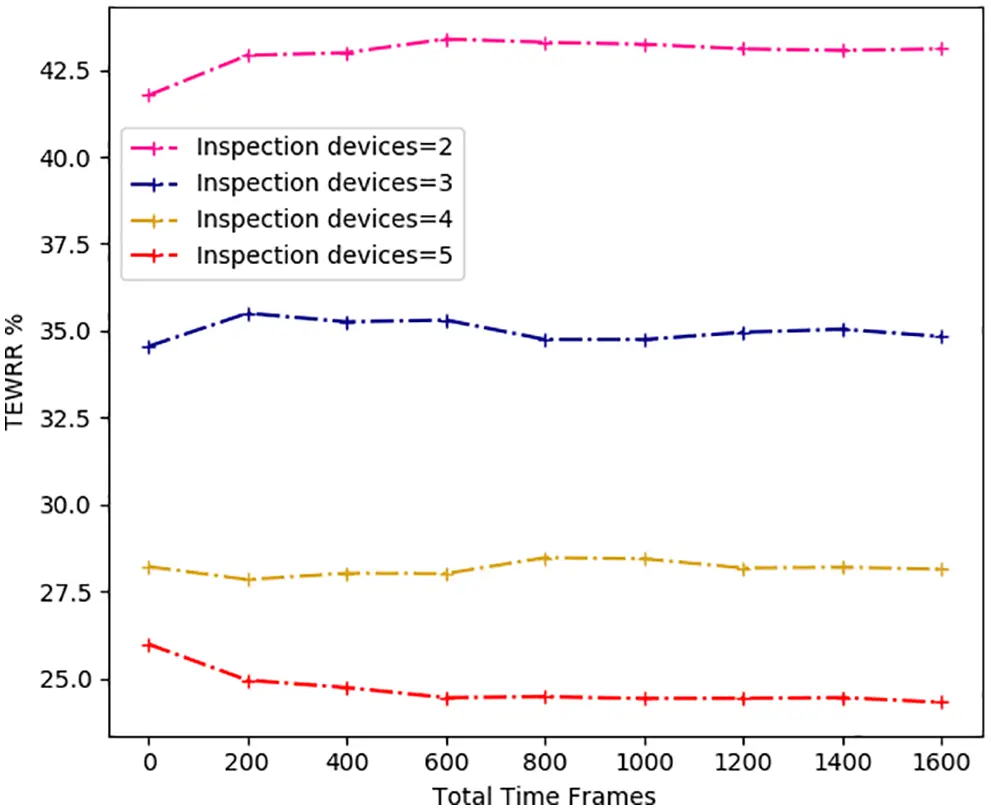Figure 8: The average TEWRR of different inspection devices N under different total time slotsFigure 9: The average TEWRR of different task number of every device I under different total time slots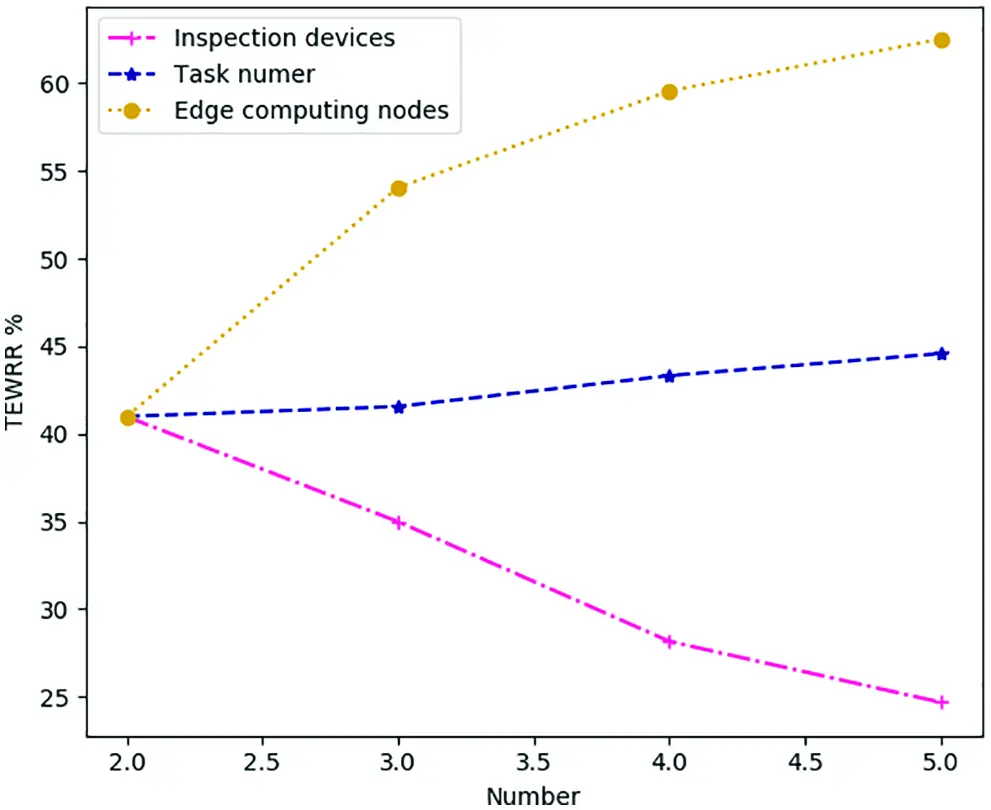Figure 10: The average TEWRR of different factor under different number in range [2, 5], step size = 1

Finally, combining Figs. 8 and 10, the correlation between the average value of TEWRR and inspection devices N is not obvious in the case of determining W and I, and the range of change is relatively small. The reason is that the total number of computing resources and tasks to be processed in each time slot in the MEC system will increase with the increase of N. At this time, the value of TEWRR is related to the task offloading strategy. The more similar the MCDDRL’s offloading strategy is to the local computing, the smaller the value of TEWRR.

6  Conclusion

Acknowledgement: We would like to thank all those who participated in the completion of the experiment and this paper.

Funding Statement: This paper is funded by the National Research & Development Project (No. 2018YFC15007005) and Sichuan Research & Development Project (No. 2020YFG0189).

Conflicts of Interest: The authors declare that they have no conflicts of interest to report regarding the present study.

## References

1. X. Chen, T. Wu, G. Sun and H. Yu, “Software-defined MANET swarm for mobile monitoring in hydropower plants,” IEEE Access, vol. 7, pp. 152243–152257, 2019.
2. C. Esposito, A. Castiglione, F. Pop and K. R. Choo, “Challenges of connecting edge and cloud computing: A security and forensic perspective,” IEEE Cloud Computing, vol. 4, no. 2, pp. 13–17, 2017.
3. K. Li, X. Tang and K. Li, “Energy-efficient stochastic task scheduling on heterogeneous computing systems,” IEEE Transactions on Parallel and Distributed Systems, vol. 25, no. 11, pp. 2867–2876, 201
4. Z. Li, J. Kang, R. Yu, D. Ye, Q. Deng et al., “Consortium blockchain for secure energy trading in industrial internet of things,” IEEE Transactions on Industrial Informatics, vol. 14, no. 8, pp. 3690–3700, 2017.
5. D. Sabella, V. Sukhomlinov, L. Trang, S. Kekki, P. Paglierani et al., “Developing software for multi-access edge computing,” ETSI White Paper, vol. 20, pp. 1–38, 2019.
6. M. Chen and Y. Hao, “Task offloading for mobile edge computing in software defined ultra-dense network,” IEEE Journal on Selected Areas in Communications, vol. 36, no. 3, pp. 587–597, 2018.
7. T. Q. Dinh, J. Tang, Q. D. La and T. Q. S. Quek, “Offloading in mobile edge computing: Task allocation and computational frequency scaling,” IEEE Transactions on Communications, vol. 65, no. 8, pp. 3571–3584, 201
8. L. Tong, Y. Li and W. Gao, “A hierarchical edge cloud architecture for mobile computing,” in Proc. IEEE International Conference on Computer Communications, San Francisco, SF, USA, vol. 2016, pp. 1–9, 2016.
9. X. Lin, Y. Wang, Q. Xie and M. Pedram, “Task scheduling with dynamic voltage and frequency scaling for energy minimization in the mobile cloud computing environment,” IEEE Transactions on Services Computing, vol. 8, no. 2, pp. 175–186, 2014.
10. L. Huang, X. Feng, A. Feng, Y. Huang and L. P. Qian, “Distributed deep learning-based offloading for mobile edge computing networks,” Mobile Networks and Applications, vol. 23, pp. 1–8, 2018.
11. S. Bi and Y. J. Zhang, “Computation rate maximization for wireless powered mobile-edge computing with binary computation offloading,” IEEE Transactions on Wireless Communications, vol. 17, no. 6, pp. 4177–4190, 2018.
12. Y. Mao, J. Zhang and K. B. Letaief, “Dynamic computation offloading for mobile-edge computing with energy harvesting devices,” IEEE Journal on Selected Areas in Communications, vol. 34, no. 12, pp. 3590–3605, 2016.
13. X. Chen, L. Jiao, W. Li and X. Fu, “Efficient multi-user computation offloading for mobile-edge cloud computing,” IEEE/ACM Transactions on Networking, vol. 24, no. 5, pp. 2795–2808, 2015.
14. S. Long, W. Long, Z. Li, K. Li, Y. Xia et al., “A game-based approach for cost-aware task assignment with QoS constraint in collaborative edge and cloud environments,” IEEE Transactions on Parallel and Distributed Systems, vol. 32, no. 7, pp. 1629–1640, 2020.
15. H. Guo and J. Liu, “Collaborative computation offloading for multiaccess edge computing over fiber–wireless networks,” IEEE Transactions on Vehicular Technology, vol. 67, no. 5, pp. 4514–4526, 2018.
16. G. Mitsis, P. A. Apostolopoulos, E. E. Tsiropoulou and S. Papavassiliou, “Intelligent dynamic data offloading in a competitive mobile edge computing market,” Future Internet, vol. 11, no. 5, pp. 118, 2019.
17. Y. Li, X. Wang, W. Fang, F. Xue, H. Jin et al., “A distributed admm approach for collaborative regression learning in edge computing,” Computers, Materials & Continua, vol. 23, no. 2, pp. 493–508, 2019.
18. T. Yang, X. Shi, Y. Li, B. Huang, H. Xie et al., “Workload allocation based on user mobility in mobile edge computing,” Journal on Big Data, vol. 2, no. 3, pp. 105–111, 2020.
19. Y. Wei, Z. Wang, D. Guo and F. R. Yu, “Deep q-learning based computation offloading strategy for mobile edge computing,” Computers, Materials & Continua, vol. 59, no. 1, pp. 89–104, 20
20. X. Chen, H. Zhang, C. Wu, S. Mao, Y. Ji et al., “Optimized computation offloading performance in virtual edge computing systems via deep reinforcement learning,” IEEE Internet of Things Journal, vol. 6, no. 3, pp. 4005–4018, 2018.
21. X. Xiong, K. Zheng, L. Lei and L. Hou, “Resource allocation based on deep reinforcement learning in IoT edge computing,” IEEE Journal on Selected Areas in Communications, vol. 38, no. 6, pp. 1133–1146, 2020.
22. J. Ren, H. Wang, T. Hou, S. Zheng and C. Tang, “Collaborative edge computing and caching with deep reinforcement learning decision agents,” IEEE Access, vol. 8, pp. 120604–120612, 2020.
23. Y. Chen, Z. Liu, Y. Zhang, Y. Wu, X. Chen et al., “Deep reinforcement learning-based dynamic resource management for mobile edge computing in industrial internet of things,” IEEE Transactions on Industrial Informatics, vol. 17, no. 7, pp. 4925–4934, 2020.
24. Y. Qian, R. Wang, J. Wu, B. Tan and H. Ren, “Reinforcement learning-based optimal computing and caching in mobile edge network,” IEEE Journal on Selected Areas in Communications, vol. 38, no. 10, pp. 2343–2355, 2020.
25. Z. Tong, X. Deng, F. Ye, S. Basodi, X. Xiao et al., “Adaptive computation offloading and resource allocation strategy in a mobile edge computing environment,” Information Sciences, vol. 537, pp. 116–131, 2020.
26. L. Huang, S. Bi and Y. -J. A. Zhang, “Deep reinforcement learning for online computation offloading in wireless powered mobile-edge computing networks,” IEEE Transactions on Mobile Computing, vol. 19, no. 11, pp. 2581–2593, 2019.
27. Y. Mao, J. Zhang, S. H. Song and K. B. Letaief, “Power-delay tradeoff in multi-user mobile-edge computing systems,” in Proc. IEEE Global Communication Conf., Washington, WA, USA, pp. 1–6, 2016.
28. Y. Mao, J. Zhang and K. B. Letaief, “Joint task offloading scheduling and transmit power allocation for mobile-edge computing systems,” in Proc. IEEE Wireless Communications & Networking Conf., San Francisco, SF, USA, pp. 1–6, 2017.
29. L. Huang, X. Feng, L. Zhang, L. Qian and L. Wu, “Multi-server multi-user multi-task computation offloading for mobile edge computing networks,” Sensors, vol. 19, no. 6, pp. 1446, 2019.This work is licensed under a Creative Commons Attribution 4.0 International License, which permits unrestricted use, distribution, and reproduction in any medium, provided the original work is properly cited.